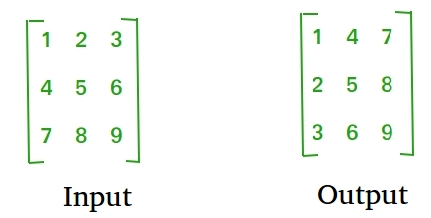# C Program to find transpose of a matrix

• Difficulty Level : Medium
• Last Updated : 16 Jun, 2022

Transpose of a matrix is obtained by changing rows to columns and columns to rows. In other words, transpose of A[][] is obtained by changing A[i][j] to A[j][i].For Square Matrix :

The below program finds transpose of A[][] and stores the result in B[][], we can change N for different dimension.

## C

 `// C Program to find``// transpose of a matrix``#include ``#define N 4` `// This function stores transpose of A[][] in B[][]``void` `transpose(``int` `A[][N], ``int` `B[][N])``{``    ``int` `i, j;``    ``for` `(i = 0; i < N; i++)``        ``for` `(j = 0; j < N; j++)``            ``B[i][j] = A[j][i];``}` `int` `main()``{``    ``int` `A[N][N] = { {1, 1, 1, 1},``                    ``{2, 2, 2, 2},``                    ``{3, 3, 3, 3},``                    ``{4, 4, 4, 4}};` `    ``int` `B[N][N], i, j;` `    ``transpose(A, B);` `    ``printf``(``"Result matrix is \n"``);``    ``for` `(i = 0; i < N; i++)``    ``{``        ``for` `(j = 0; j < N; j++)``        ``printf``(``"%d "``, B[i][j]);``        ``printf``(``"\n"``);``    ``}` `    ``return` `0;``}`

Output:

``` Result matrix is
1 2 3 4
1 2 3 4
1 2 3 4
1 2 3 4 ```

Time Complexity : O(n 2 )

Auxiliary Space : O(n 2 )

For Rectangular Matrix :

The below program finds transpose of A[][] and stores the result in B[][].

## c

 `#include ``#define M 3``#define N 4` `// This function stores transpose of A[][] in B[][]``void` `transpose(``int` `A[][N], ``int` `B[][M])``{``    ``int` `i, j;``    ``for` `(i = 0; i < N; i++)``        ``for` `(j = 0; j < M; j++)``            ``B[i][j] = A[j][i];``}` `int` `main()``{``    ``int` `A[M][N] = { {1, 1, 1, 1},``                    ``{2, 2, 2, 2},``                    ``{3, 3, 3, 3}};` `    ``// Note dimensions of B[][]``    ``int` `B[N][M], i, j;` `    ``transpose(A, B);` `    ``printf``(``"Result matrix is \n"``);``    ``for` `(i = 0; i < N; i++)``    ``{``        ``for` `(j = 0; j < M; j++)``        ``printf``(``"%d "``, B[i][j]);``        ``printf``(``"\n"``);``    ``}` `    ``return` `0;``}`

Output:

``` Result matrix is
1 2 3
1 2 3
1 2 3
1 2 3 ```

Time Complexity : O(n*m)

Auxiliary Space : O(n*m)

Please refer complete article on Program to find transpose of a matrix for more details!

My Personal Notes arrow_drop_up